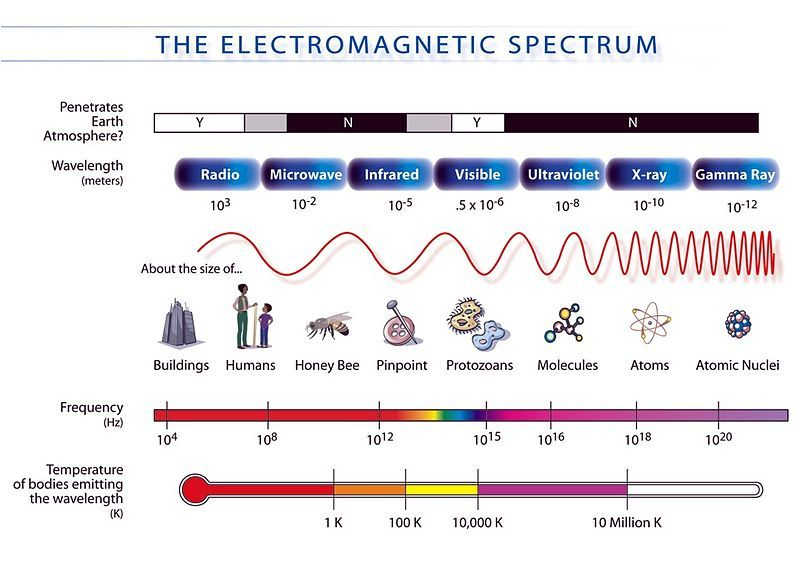Home Practice
For learners and parents For teachers and schools
Textbooks
Full catalogue
Pricing SupportLog in

We think you are located in United States. Is this correct?

# 11.3 Electromagnetic spectrum

EM radiation is classified into types according to the frequency of the wave: these types include, in order of increasing frequency, radio waves, microwaves, infrared radiation, visible light, ultraviolet radiation, X-rays and gamma rays.

Table 11.1 lists the wavelength and frequency ranges of the divisions of the electromagnetic spectrum.

 Category Range of Wavelengths (nm) Range of Frequencies (Hz) gamma rays $$< 1$$ $$> \text{3} \times \text{10}^{\text{17}}$$ X-rays $$1–10$$ $$\text{3} \times \text{10}^{\text{16}} – \text{3} \times \text{10}^{\text{17}}$$ ultraviolet light $$10–400$$ $$\text{7,5} \times \text{10}^{\text{14}} – \text{3} \times \text{10}^{\text{16}}$$ visible light $$400–700$$ $$\text{4,3} \times \text{10}^{\text{14}} – \text{7,5} \times \text{10}^{\text{14}}$$ infrared $$700 – \text{10}^{\text{5}}$$ $$\text{3} \times \text{10}^{\text{12}} – \text{4,3} \times \text{10}^{\text{14}}$$ microwave $$\text{10}^{\text{5}} – \text{10}^{\text{8}}$$ $$\text{3} \times \text{10}^{\text{9}} – \text{3} \times \text{10}^{\text{12}}$$ radio waves $$> \text{10}^{\text{8}}$$ $$< \text{3} \times \text{10}^{\text{9}}$$

Table 11.1: Electromagnetic spectrum

Examples of some uses of electromagnetic waves are shown in Table 11.2.

 Category Uses gamma rays used to kill the bacteria in marshmallows and to sterilise medical equipment X-rays used to image bone structures ultraviolet light bees can see into the ultraviolet because flowers stand out more clearly at this frequency visible light used by humans to observe the world infrared night vision, heat sensors, laser metal cutting microwave microwave ovens, radar radio waves radio, television broadcasts

Table 11.2: Uses of EM waves

Textbook Exercise 11.1

Arrange the following types of EM radiation in order of increasing frequency: infrared, X-rays, ultraviolet, visible, gamma.

Solution not yet available

Calculate the frequency of an EM wave with a wavelength of $$\text{400}$$ $$\text{nm}$$.

Solution not yet available

Give an example of the use of each type of EM radiation, i.e. gamma rays, X-rays, ultraviolet light, visible light, infrared, microwave and radio and TV waves.

Solution not yet availableFigure 11.2: The electromagnetic spectrum as a function of frequency. The different types according to wavelength are shown as well as everyday comparisons.

EM radiation in the visible part of the spectrum is scattered off all of the objects around us. This EM radiation provides the information to our eyes that allows us to see. The frequencies of radiation the human eye is sensitive to constitute only a very small part of all possible frequencies of EM radiation. The full set of EM radiation is called the electromagnetic spectrum. To simplify things the EM spectrum divided into sections (such as radio, microwave, infrared, visible, ultraviolet, X-rays and gamma-rays).

The EM spectrum is continuous (has no gaps) and infinite. Due to technological limitations, we can only use electromagnetic radiation with wavelengths between $$\text{10}^{-\text{14}}$$ $$\text{m}$$ and $$\text{10}^{\text{15}}$$ $$\text{m}$$.